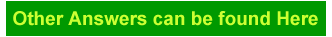## How to write a formula in excel in Excel

How to write a formula in Excel?

How to add, substract, multiply or divide two numbers in Excel?

How do you calculate in Excel?

How do I create a function in Excel?

To make a calculation in Excel it is all about playing with cells (A1, B4, G11, ...) by creating an formula in a destination cell.

Like like writing in B2 the formula = (A1 + B1) * G11

(for formulas, depending on your country, you might have to change ; with , or the opposite